Courses

# Sampling & Reconstruction of Band Limited Signals Notes | EduRev

## Electrical Engineering (EE): Sampling & Reconstruction of Band Limited Signals Notes | EduRev

The document Sampling & Reconstruction of Band Limited Signals Notes | EduRev is a part of the Electrical Engineering (EE) Course Signals and Systems.
All you need of Electrical Engineering (EE) at this link: Electrical Engineering (EE)

Sampling and Reconstruction of Band-Limited Signals

Band-limited signals: A Band-limited signal is one whose Fourier Transform is non-zero on only a finite interval of the frequency axis.
Specifically, there exists a positive number B such that X(f) is non-zero only in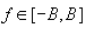. B is also called the Bandwidth of the signal.
To start off, let us first make an observation about the class of Band-limited signals.

Lets consider a Band-limited signal x(t) having a Fourier Transform X(f). Let the interval for which X(f) is non-zero be -B f B .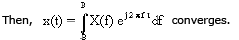The RHS of the above equation is differentiable with respect to t any number of times as the integral is performed on a bounded domain and the integrand is differentiable with respect to t. Further, in evaluating the derivative of the RHS, we can take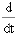inside the integral.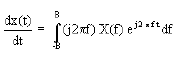In general,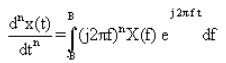This implies that band limited signals are infinitely differentiable, therefore, very smooth .
We now move on to see how a Band-limited signal can be reconstructed from its samples.

Reconstruction of Time-limited Signals

Consider first a signal y(t) that is time-limited, i.e. it is non-zero only in [-T/2, T/2].
Its Fourier transform Y(f) is given by: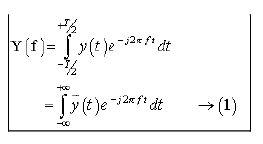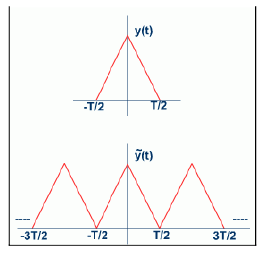Where is the periodic extension of y(t) as shown

Now, Recall that the coefficients of the Fourier series for a periodic signal x(t) are given by :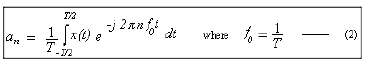Comparing (1) and (2), you will find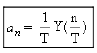That is, the Fourier Transform of the periodic signal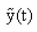is nothing but the samples of the original transform.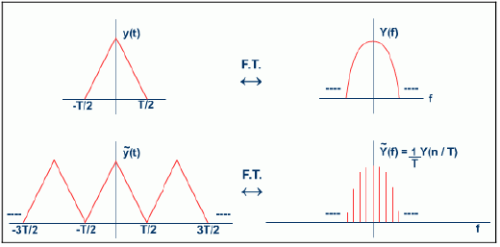Therefore, given that; y(t) is time-limited in [-T/2, T/2] and periodic, the entire information about y(t) is contained in just equispaced samples of its Fourier transform! It is the dual of this result that is the basis of Sampling and Reconstruction of Bandlimited signals :-
Knowing the Fourier transform is limited to, say [-B, B], the entire information about the transform (and hence the signal) is contained in just uniform samples of the (time) signal !

Reconstruction of Band-limited signals

Let us now apply the dual reasoning of the previous discussion to Band-limited signals. x(t) is Band-limited, with its Fourier transform X(f) being non-zero only in [-B, B]. The dual reasoning of the discussion in previous slide will imply that we can reconstruct X(f) perfectly in [-B, B] by using only the samples x( n / 2B ). Let's see how.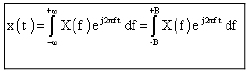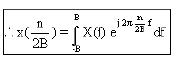This time,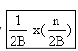is the  -nthFourier series co-efficient of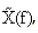, the periodic extension of X(f).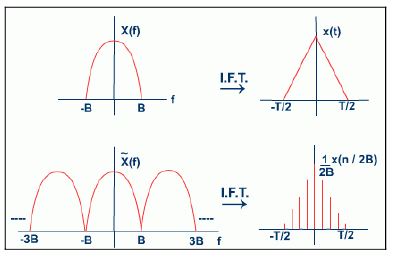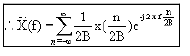(Fourier series in f -- fundamental period is 2B and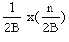is the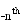Fourier series coefficient)'

What is the Fourier inverse of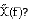The Fourier inverse of  is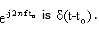. Therefore, the Fourier inverse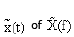is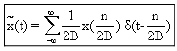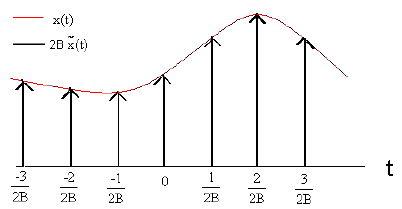Thus we see that if we multiply the original Band-limited signal with a periodic train of impulses (period 1/2B, with impulse at the origin of strength 1/2B ) we obtain a signal whose Fourier transform is a periodic extension of the original spectrum. So how does one retrieve the original signal from ? We need a mechanism that will blank out the spectrum of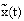in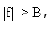, i.e: multiply the spectrum with :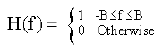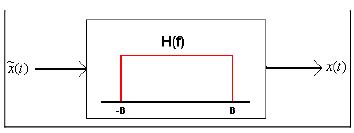In other words, we need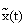to feed  to an LSI system, the Fourier transform of whose impulse response is the above function (recall the convolution theorem), i.e: one whose impulse response is: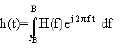An LSI system with above type of impulse response is called an Ideal Low Pass Filter .

The Sampling Theorem

On the basis of our discussion so far, we may state formally the Sampling Theorem.

Shannon-Whiltaker-Nyquist Sampling Theorem:

A band-limited signal with band-width B may be reconstructed perfectly from its samples, if the signal is sampled uniformly at a rate greater than 2B.

Is it essential for the sampling rate to be greater than 2B, or is it acceptable to have a sampling rate of exactly 2B?
What will happen if the value of X(f) at -B and B are not zero?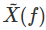will have values at B and -B different from those of X(f) (due to the periodic expension). Thus the transform of the output of the ideal low pass filter will not match that of the original signal at -B and B.  While finite, point mismatches in the transform will not matter, problems arise if X(f) has impulses at B or -B. Then, the output of the ideal low pass filter will be different from the original signal.

For example, consider sin(t). It has a bandwidth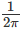. Say we sample the signal at a rate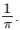What happens to all our samples? The signal has value zero at all multiples of π! You can't possibly reconstruct the signal from these samples. What went wrong? Lets look at the Fourier Transform involved: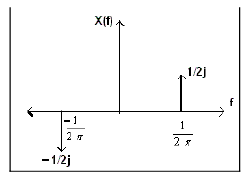Note that the periodic extension (taking period to be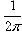) of this signal is identically zero. Thus an ideal low pass filter cannot retrieve this spectrum from its periodic extension.

This is why the Sampling theorem says one must use a sampling rate greater than 2B, where B is the Bandwidth of the signal. Say we sample at a rate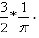What is the Fourier transform of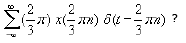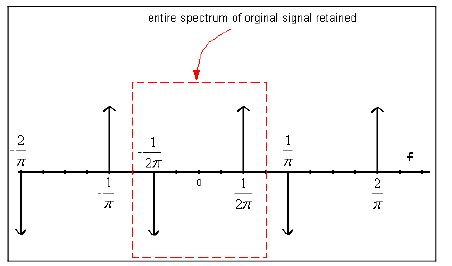Now, an appropriate Low-pass filter can give us back the original signal !

Conclusion:

In this lecture you have learnt:

• Band-limited signals are infinitely differentiable and very smooth.
• Given that 'x(t)' is Band-limited with its Fourier transform 'X(f)' being non-zero only in [-B,B] , we can say that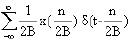has a
spectrum that is the periodic extension of 'X(f)' with period 2B

By passing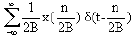through an appropriate Ideal Low-pass filter one can obtain back 'x(t)'.

Shannon-Whiltaker-Nyquist Sampling Theorem:

A band-limited signal with band-width 'B' may be reconstructed perfectly from its samples, if the signal is sampled at a rate greater than '2B'.

The document Sampling & Reconstruction of Band Limited Signals Notes | EduRev is a part of the Electrical Engineering (EE) Course Signals and Systems.
All you need of Electrical Engineering (EE) at this link: Electrical Engineering (EE)Use Code STAYHOME200 and get INR 200 additional OFF Use Coupon Code
All Tests, Videos & Notes of Electrical Engineering (EE): Electrical Engineering (EE)

### Top Courses for Electrical Engineering (EE)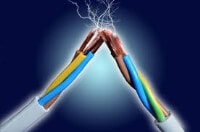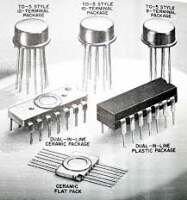## Signals and Systems

40 videos|40 docs|25 tests

### Top Courses for Electrical Engineering (EE)Track your progress, build streaks, highlight & save important lessons and more!

,

,

,

,

,

,

,

,

,

,

,

,

,

,

,

,

,

,

,

,

,

;